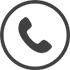1300 556 601

# Hazardous Area Intrinsically Safe Isolators

Grid List
Currently Filtered By...
##### Filter By
Currently Filtered By...
There are no filter options available
Increase value Decrease value
Increase value Decrease value
Increase value Decrease value
Increase value Decrease value
Increase value Decrease value
Increase value Decrease value
Increase value Decrease value
Increase value Decrease value
Increase value Decrease value
Increase value Decrease value
Increase value Decrease value
Increase value Decrease value
Increase value Decrease value
Increase value Decrease value
Increase value Decrease value
Increase value Decrease value
Increase value Decrease value
Increase value Decrease value
Increase value Decrease value
Increase value Decrease value
##### Products to compare:
Comparing Products